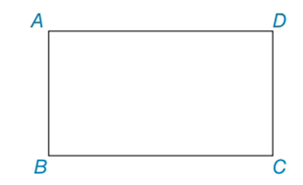Chapter 4.3, Problem 9E### Elementary Geometry for College St...

6th Edition
Daniel C. Alexander + 1 other
ISBN: 9781285195698

#### Solutions

Chapter
Section### Elementary Geometry for College St...

6th Edition
Daniel C. Alexander + 1 other
ISBN: 9781285195698
Textbook Problem
1 views

# In Exercises 8 to 10, use the properties of rectangles to solve each problem. Rectangle ABCD is shown in the figure.In Exercise 9 and 10, see the figure for Exercise 8.Exercises 8 - 10 Given: AB = 2x + 7 and BC = 3x + 4, and CD = 3x + 2 Find: x and DA

To determine

To find:

x and DA of the given rectangle.

Explanation

The basic property of rectangle:

1) All the properties of a parallelogram apply (the ones that matter here are parallel sides, opposite sides are congruent, and diagonals bisect each other).

2) All angles are right angles by definition.

3) The diagonals are congruent.

Calculation:

Given: AB = 2x + 7 and BC = 3x + 4, and CD = 3x + 2

Consider the rectangle.

Now we have to find x and DA of the given rectangle.

To find x:

From the property, opposite sides are congruent in rectangle.

So, AB = CD

2x+7=3x+23x

### Still sussing out bartleby?

Check out a sample textbook solution.

See a sample solution

#### The Solution to Your Study Problems

Bartleby provides explanations to thousands of textbook problems written by our experts, many with advanced degrees!

Get Started